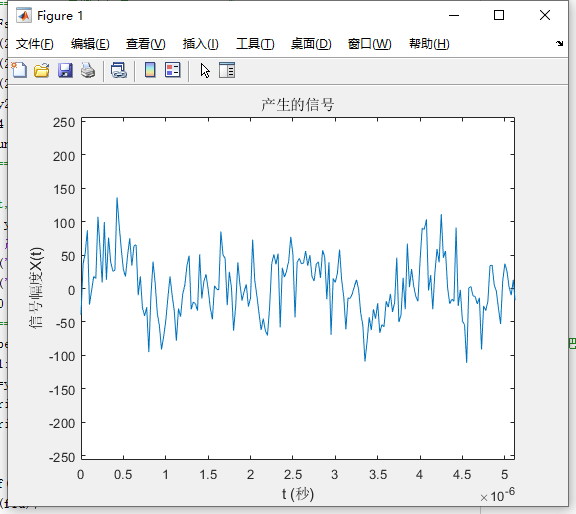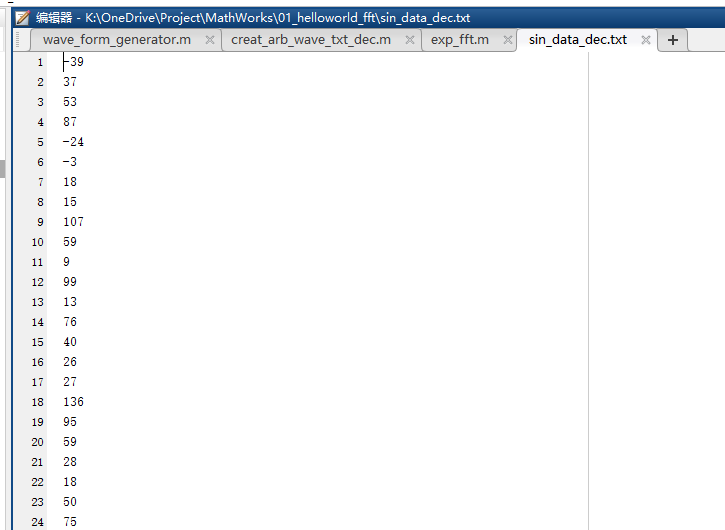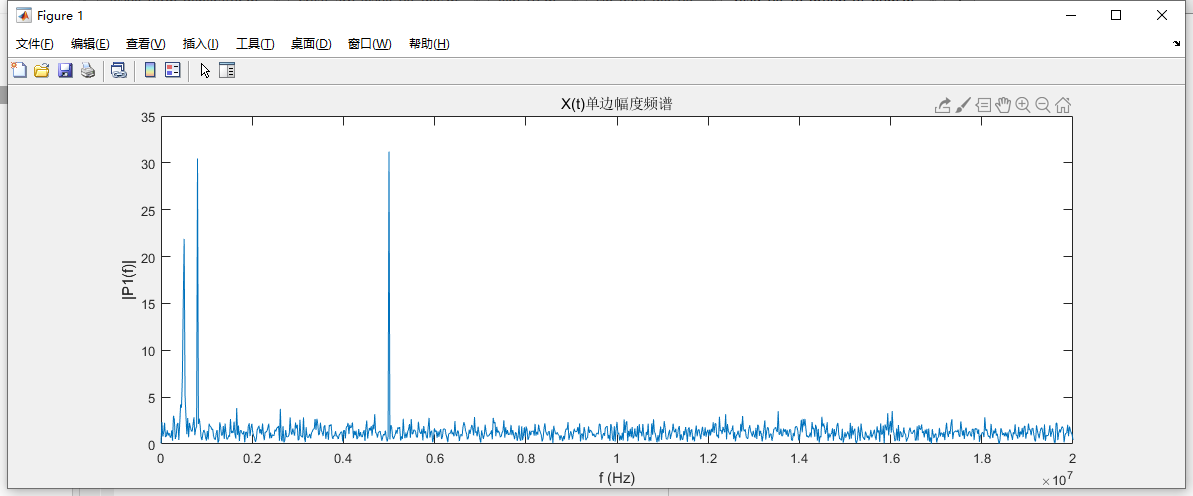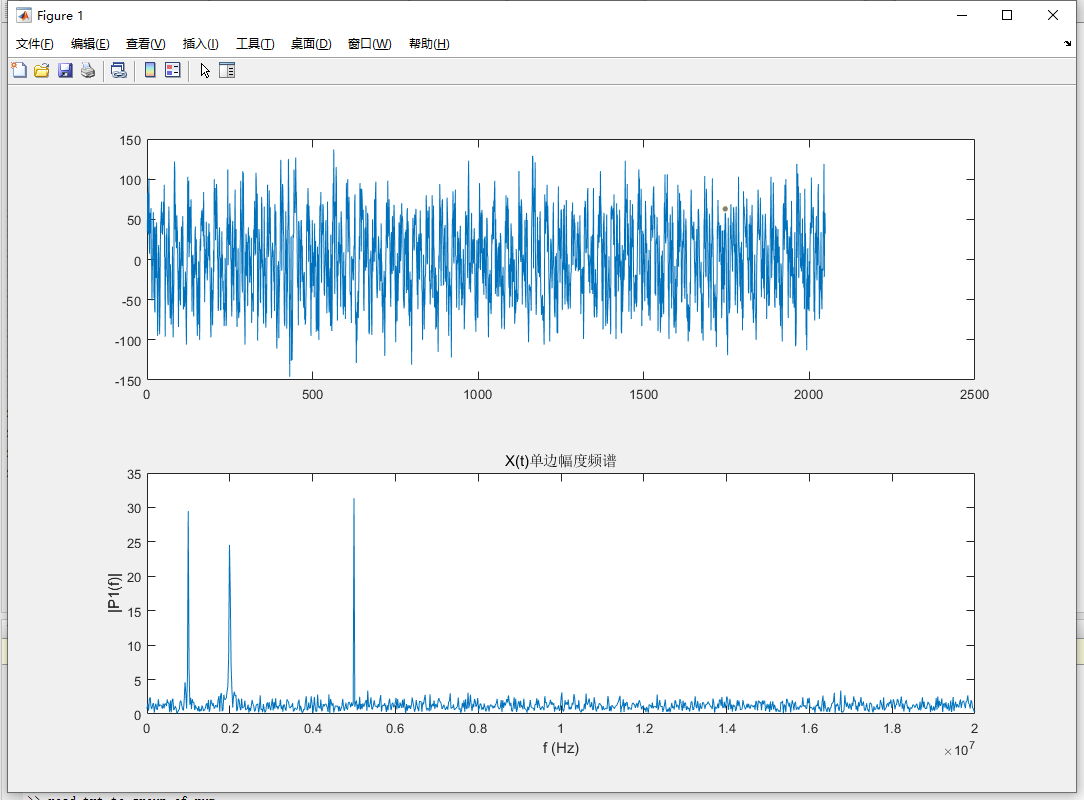• 使用Matlab读取txt数据（将多个txt文件中的数据合并）file = dir('result\*.txt'); %读取文件夹目录 out = []; for i = 1 : length(file) in = load(['result\',file(i).name]);%依次载入文件(仅限于数字) out = ...
使用Matlab读取txt数据（将多个txt文件中的数据合并）
file = dir('result\*.txt');  %读取文件夹目录
out = [];
for i = 1 : length(file)
out = [out, in'];  %合并txt中的内容，存入矩阵中
end
fid = fopen('out.txt','wt');  %将文件读出
fprintf(fid,['%g  ', '%g','\n'],out);   %格式输出
%fprintf(fid,[repmat('%g ',1,size(out,2)), '\n'],out);
fclose(fid); 
另一种读入的方式：
all_name = fopen('All.txt');
line = fgetl(all_name);%逐行读入（包括数字，字符串）
count = 1;
while ischar(line)
str{count,:} = line;  %按行存入cell中
line = fgetl(all_name);
count = count + 1;
end
fclose(all_name);
展开全文• matlab使用的版本：matlab 2018A txt文件内的数据格式： 00 00 00 00 00 00 00 00 00 00 00 00 00 00 00 等多维数组。 matlab代码： clc;clear;close all;a temp = textread('a.txt','%s'); n=length(temp(:)...
matlab使用的版本：matlab 2018A
txt文件内的数据格式：
00 00 00 00 00
00 00 00 00 00
00 00 00 00 00
等多维数组。
matlab代码：
clc;clear;close all;a temp = textread('a.txt','%s'); n=length(temp(:));%计算数组的长度 a=cell2mat(temp); b = hex2dec(a); d3 = reshape(b,3,11520); d12 = reshape(b,12,2880);
%------------  产生一个 24 bit 的mif 文件  fid=fopen('dsc_data_24bit.mif','w');%打开待写入的.mif文件 fprintf(fid,'WIDTH=24;\n');%写入存储位宽24位 fprintf(fid,'DEPTH=8192;\n');%写入存储深度8192 fprintf(fid,'ADDRESS_RADIX=UNS;\n');%写入地址类型为无符号整型 fprintf(fid,'DATA_RADIX=HEX;\n');%写入数据类型为16进制 fprintf(fid,'CONTENT BEGIN\n');%起始内容  for i = 0:8191           fprintf(fid,'\t%d\t:',i);         fprintf(fid,'\t%x',d3(1,i+1));         fprintf(fid,'%x',d3(2,i+1));         fprintf(fid,'%x',d3(3,i+1));         fprintf(fid,';\n'); end fprintf(fid,'END;\n'); fclose(fid);%关闭文件

mif的文件就是一个24bit数据宽度的数据。
展开全文• Porject_Kiwi 离线式数字信号处理（一） 使用Matlab读取TXT数据文件并做FFT分析 在我群项目Project_Kiwi中，LTC2208的评估已经圆满完成，现在开始下一步的工作。 今天要来测试的，是使用matlab对数据进行简单处理。 ...
Porject_Kiwi
离线式数字信号处理（一）
使用Matlab读取TXT数据文件并做FFT分析
在我群项目Project_Kiwi中，LTC2208的评估已经圆满完成，现在开始下一步的工作。 今天要来测试的，是使用matlab对数据进行简单处理。因为考虑到目前FPGA到PC的数据链路仍然还没有搭建，所以暂时先搭建一个离线式测试平台。等待我们的算法逐渐完整，可以正常运行时，再来调试其到PC的链路，可能是USB，甚至可能是PCIE（先画个饼）
萌新起步，大佬勿喷。
第一步的想法是使用SD卡来存取采集的数据，使用FATFS可以迅速在各种平台移植（STM32等ARM平台），SD卡也可以存储大量的数据，随后可以接入电脑使用matlab等工具对数据进行读取并作进一步的处理。

SD卡

STM32, FPGA

PC

为了测试matlab程序，我们需要先生成一个.txt文件，STM32的程序已经叫学弟在写了，我们先用Matlab生成一个先跑着。（学弟好像还在调车） 我的程序基于这位大佬的程序进行轻微修改
%=============设置系统参数==============%
f1=5e6;        %设置波形频率
f2=500e3;
f3=800e3;
Fs=40e6;        %设置采样频率
L=2048;         %数据长度
N=8;           %数据位宽
%=============产生输入信号==============%
t=0:1/Fs:(1/Fs)*(L-1);
y1=sin(2*pi*f1*t);
y2=sin(2*pi*f2*t);
y3=sin(2*pi*f3*t);
y4=y1+y2+y3;
y5 = y4 + 1*randn(size(t));
y_n=round(y5*(2^(N-3)-1));      %N比特量化;如果有n个信号相加，则设置（N-n）
%=================画图==================%
a=10;           %改变系数可以调整显示周期
%stem(t,y_n);
plot(t,y_n);
axis([0 L/Fs/a -2^N 2^N]);      %显示
%=============写入外部文件==============%
fid=fopen('D:\OneDrive\Project\MathWorks\01_helloworld_fft\sin_data_dec.txt','w');    %把数据写入sin_data.txt文件中，如果没有就创建该文件
for k=1:length(y_n)
tb=y_n(k);
fprintf(fid,'%d',tb);
fprintf(fid,'\r\n');
end

fprintf(fid,';');
fclose(fid);


运行结果如图所示运行得到的TXT文件如图。好了，现在我们有了测试用的TXT文件，就可以开始运行处理程序了。 目前处理程序还非常的简陋，之后会继续往里面添加更多的东西。
%读取txt格式的波点数据到数组并绘图

%信号的参数
Fs = 40e6;            % 采样频率
T = 1/Fs;             % 采样周期（周期=1/频率）
L = 2048;             % 信号长度，2048
t = (0:L-1)*T;        % 时间向量

wave_data=importdata('K:\OneDrive\Project\MathWorks\01_helloworld_fft\sin_data_dec.txt');
subplot(2,1,1);plot(wave_data) ;
Y = fft(wave_data);

P2 = abs(Y/L);
P1 = P2(1:L/2+1);
P1(2:end-1) = 2*P1(2:end-1);

f = Fs*(0:(L/2))/L;

subplot(2,1,2);plot(f,P1) ;
title('X(t)单边幅度频谱');
xlabel('f (Hz)');
ylabel('|P1(f)|');

运行结果如图：可以在单边幅度谱中看到我们刚才生成的500Hz、800Hz、5MHz的三个峰值。以上desu。
展开全文数字信号处理
在使用Matlab处理数据时，我们经常需要读取txt文档，可以使用Matlab中强大的textread函数。

它的基本语法是：

filename就是文件名， format就是要读取的格式，A,B,C就是从文件中读取到的数据。中括号里面变量的个数必须和format中定义的个数相同。 如果每N行相同格式的数据，可采用

举例如下：
例1：myfile.txt 中的内容如下：    1 2 3 4    5 6 7 8    9 10 11 12相应的语句为：filename = '.\myfile.txt';[data1,data2,data3,data4]=textread(filename,'%n%n%n%n');或者为

例2：myfile.txt 中的内容如下：    1, 2, 3, 4    5, 6, 7, 8    9, 10, 11, 12相应的语句为：filename = '.\myfile.txt';[data1,data2,data3,data4]=textread(filename,'%n%n%n%n',
'
delimiter
'
,
'
,
'
);这里
delimiter指出分隔符，读数据的时候会自动跳过分隔符。

例3：myfile.txt 中的内容如下：    % this a comment    1, 2, 3, 4    5, 6, 7, 8    9, 10, 11, 12相应的语句为：filename = '.\myfile.txt';[data1,data2,data3,data4]=textread(filename,'%n%n%n%n',
'
delimiter
'
,
'
,
'
,
'
headerlines
'
, 1
myfile.txt 中的内容如下：

Sally Level1 12.34 45 Yes
相应语句为：
filename = '.\myfile.txt';
[names, types, x, y, answer] = textread(filename , '%s %s %f %d %s', 1)
②如果要忽略Level，只读取后面的数字：[names, levelnum, x, y, answer] = textread(filename , ... '%s Level%d %f %d %s', 1)

例5：myfile.txt 中的内容如下
1,2,3,4,,6
7,8,9,,11,12
想用nan替代为空的部分。语句如下:data = textread('myfile.txt', 'delimiter', ',', 'emptyvalue', NaN)。

例6： myfile.txt 中的内容如下
Sally    Type1 12.34 45 Yes
Joe      Type2 23.54 60 No
Bill     Type1 34.90 12 No
如果只想读第一列，其余的跳过：
filename = '.\myfile.txt';
%[^...] - reads characters not matching characters between the brackets until first matching character
%[^\n] 就是一直读到行尾
%*[^\n] 就是从当前直接跳到行尾
例7: myfile.txt 中的内容如下
Location;date;discharge
Lobith;1989-01-01;00:00;2801
Lobith;1989-01-02;00:00;2619
语法如下：
[Location,... % 1
yyyy              ,... % 2a datestring year
mm                ,... % 2b datestring month
dd                ,... % 2c datestring day
HH                ,... % 3a timestring hour
MM                ,... % 3b timestring minute
discharge] =  ...
'delimiter'  ,';');
注意：textread不用先fopen那个文件，适用于格式统一的txt文件的一次性大批量读取。textread读取某个文件后，下次再用textread读取这个文件时，还是会从文件头开始读取。
展开全文• matlab读取txt文件 对于纯数据文件的txt文件，直接使用load函数 load data.txt %import the txt file data in the workspace 对于含有字符的文本文件，使用importdata()函数 clear; close all; clc; %% ...
• matlab读取txt文件并作图，txt为光谱仪测量的反射率数据，附上代码和反射率数据。
• 使用matlab读取txt文本中的数据，数据最好有一定的规律，我们可以使用函数importdata来导入数据。 下面使用一个例子来说明该函数的使用： start_path = 'C:\'; % 设置默认文件夹 [filename, pathname] = ...
• 有个朋友需要我帮忙写个matlab脚本读取100个txt文档的实验数据，这些文档的结构相同，分为四列，从第一列到第四列依次是时间、位置、速度、加速度。读取完数据之后需要对数据进行处理，具体的处理方式是：提取以0....数据转换 提取特征数据
• MATLAB读取和写入txt文件 ...txt文件中既有空格又有tab键，请问怎么正确读取txt里面内容？ https://www.ilovematlab.cn/thread-529920-1-1.html MATLAB读取和写入Excel文件 1. 读取Excel文件 ...
• 关于MATLAB读取txt文件的方法 一、如何读取一个文档下，多个txt文件？ 一般读取的文件，命名都具有一定的规律，除去相同部分，剩下的变化按照时间、序号、某一特定的标志符等进行排列。 下面我们举个例子来操作。 ...
• 如图所示， 此类型的变量不支持使用点进行索引 这个程序之前都是好的，没有做任何改动，早上运行过，今晚就突然报错请问这是为什么，谢谢 我发现我的工作区textData显示只有元胞数组，没有data数据 读取数据不...c++ 开发语言 c语言 c++ 开发语言
• 文章参考:... 笔记留作个人科研使用,如有转载不允许,请告知,立刻删除. 文件读取方法 目标：读取每一行数字数目不同的纯文本文件。形如： 9 289734689 002874...
• %% 格式化文本的读操作   %只读形式打开txt文件 file_t = fopen('mytxt.txt','r'); %以十进制读取,且读取的数据自动排成一列，排的顺序为：先从第一行左边...%% 使用textscan读取多列数据 file_t = fop...
• 幅值为5、频率为10Hz的正弦信号，并写入数据文件MySin.txt，并读取文本文件MySin.txt 中的数据文件，进行绘图.% Applying the Mysin function to generate a sine waveform...with amplitude and frequency are 5 ...
• ## MATLAB读取txt 指定行

千次阅读 2019-12-13 17:03:28
fid = fopen(FileText); % 文件名 % 方案一 lines= textscan(fid,'%[^\n]',20,'Headerlines',5); % 跳过5行，读下面的20行 disp(lines{1}) % 方案二 for i= 1:20 tline = fgetl(fid); if i>.........

# 利用matlab读取txtmatlab 订阅